# Lookup & Reference Functions

Learn all about Excel’s lookup & reference functions, such as VLOOKUP, HLOOKUP, MATCH, INDEX and CHOOSE.

### Vlookup

The VLOOKUP (Vertical lookup) function looks for a value in the leftmost column of a table, and then returns a value in the same row from another column you specify.

1. Insert the VLOOKUP function shown below.Explanation: the VLOOKUP function looks for the ID (104) in the leftmost column of the range \$E\$4:\$G\$7 and returns the value in the same row from the third column (third argument is set to 3). The fourth argument is set to FALSE to return an exact match or a #N/A error if not found.

2. Drag the VLOOKUP function in cell B2 down to cell B11.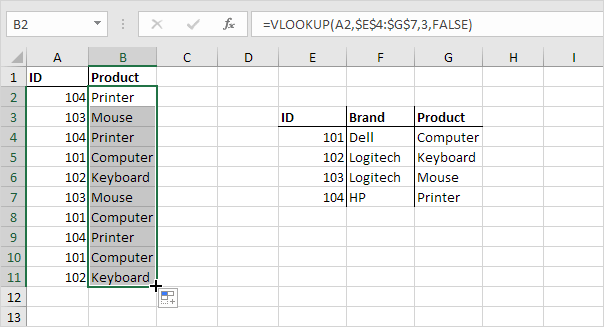Note: when we drag the VLOOKUP function down, the absolute reference (\$E\$4:\$G\$7) stays the same, while the relative reference (A2) changes to A3, A4, A5, etc. Visit our page about the VLOOKUP function for much more information and many examples.

### Hlookup

In a similar way, you can use the HLOOKUP (Horizontal lookup) function.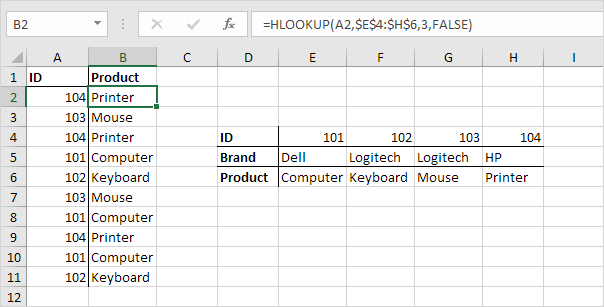Note: if you have Excel 365 or Excel 2021, use XLOOKUP instead of HLOOKUP to perform a horizontal lookup.

### Match

The MATCH function returns the position of a value in a given range.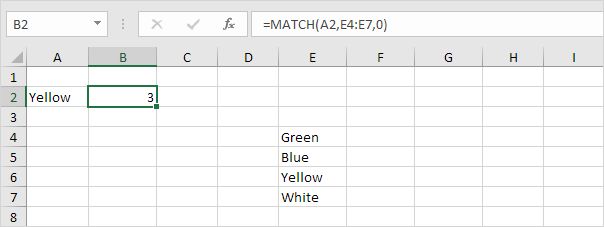Explanation: Yellow found at position 3 in the range E4:E7. The third argument is optional. Set this argument to 0 to return the position of the value that is exactly equal to lookup_value (A2) or a #N/A error if not found. Use INDEX and MATCH in Excel and impress your boss.

### Index

The INDEX function below returns a specific value in a two-dimensional range.Explanation: 92 found at the intersection of row 3 and column 2 in the range E4:F7.

The INDEX function below returns a specific value in a one-dimensional range.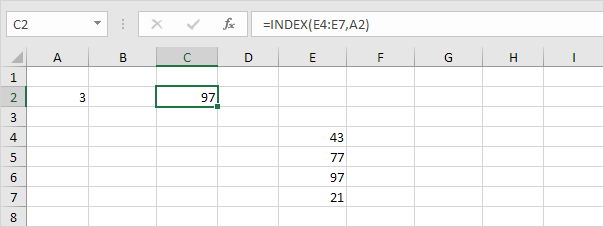Explanation: 97 found at position 3 in the range E4:E7. Use INDEX and MATCH in Excel and impress your boss.

### Choose

The CHOOSE function returns a value from a list of values, based on a position number.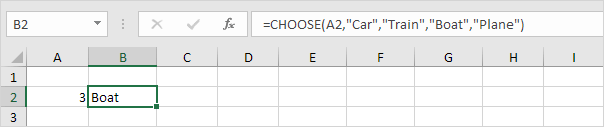Explanation: Boat found at position 3.

Previous articleXML in Excel
Next articleArray Formulas in Excel